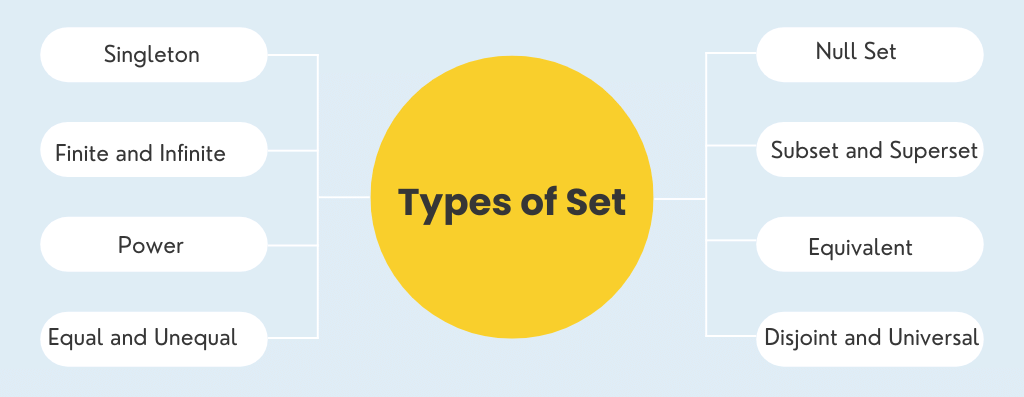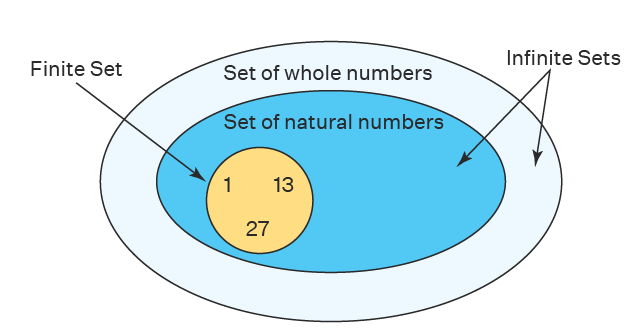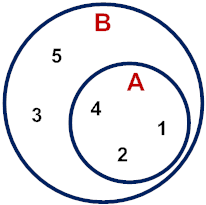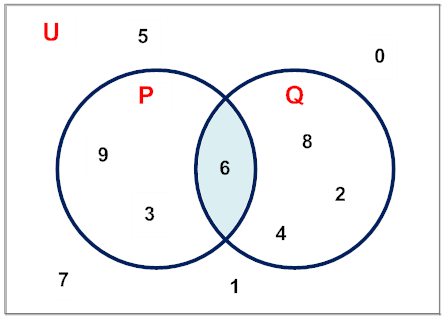Types of Set

# Types of Set - Notes | Study Algebra for IIT JAM Mathematics - Mathematics

 Table of contentsDefinition - Set theoryTypes of SetSolved Examples for You1 Crore+ students have signed up on EduRev. Have you?

Definition - Set theory

The set is a well-defined collection of definite objects of perception or thought.
• A set may also be thought of as grouping together of single objects into a whole.
• The objects should be distinct from each other and they should be distinguished from all those objects that do not form the set under consideration. Hence a set may be a bunch of grapes, a tea set or it may consist of geometrical points or straight lines.
• A set is defined as an unordered collection of distinct elements of the same type where the type is defined by the writer of the set.
• Generally, a set is denoted by a capital symbol and the master or elements of a set are separated by commas and enclosed in { }. More symbols used in the set are given below:
1 ∈ A means 1 belongs to set A
1 ∉ A  means 1 does not belong to A

Types of Set
There are many types of set in the set theory:1. Singleton Set

If a set contains of only one element it is called to be a singleton set.

Examples:

• The sets given by  {1}, {0}, {a} are all consisting of only one element and therefore are singleton sets.
• E = {x : x ϵ N and x3 = 27} is a singleton set with a single element {3}
• W = {v: v is a vowel letter and v is the first alphabet of English} is also a singleton set with just one element {a}.

2. Finite Set

As the name implies, a set with a finite or exact countable number of elements is called a finite set. If the set is non-empty, it is called a non-empty finite set.

Examples:

• A = {a, e, i, o, u} is a finite set because it represents the vowel letters in the English alphabetical series.
• B = {x : x is a number appearing on a dice roll} is also a finite set because it contains – {1, 2, 3, 4, 5, 6} elements.

3. Infinite Set

A set with an infinite number of elements is called an infinite set. In other words, if a given set is not finite, then it will be an infinite set.

Examples:

• C = {p: p is a prime number} is an infinite set.
• D = {k: k is a real number} is also an infinite set.Finite and Infinite Sets

4. Equal and Unequal Sets

Two sets X and Y are said to be equal if they have exactly the same elements (irrespective of the order of appearance in the set). Equal sets are represented as X = Y. Otherwise, the sets are referred to as unequal sets, which are represented as X ≠ Y.

• If X = {a, e, i, o, u} and H = {o, u, i, a, e} then both of these sets are equal.
• If C = {1, 3, 5, 7} and D = {1, 3, 5, 9} then both of these sets are unequal.
• If A = {b, o, y} and B = {b, o, b, y, y} then also A = B because both contain same elements.

Question for Types of Set
Try yourself:{x: x is a real number between 1 and 2} is an ______

5. Null Set/ empty Set

Any Set that does not contain any element is called the empty or null or void set. The symbol used to represent an empty set is – {} or φ (called phi)

Examples:

• Let A = {x : 9 < x < 10, x is a natural number} will be a null set because there is NO natural number between numbers 9 and 10. Therefore, A = {} or φ
• Let W = {d: d > 8, d is the number of days in a week} will also be a void set because there are only 7 days in a week.

6. Subset and Super Set

A set S is said to be a subset of set T if the elements of set S belong to set T, or you can say each element of set S is present in set T. Subset of a set is denoted by the symbol (⊂) and written as S ⊂ T and  T is the superset of set S(T ⊇ S).

Example:

Example: A = {1,2,3} B = {1,2,3,4,5,6}
B, since all the elements in set A are present in set B.
B ⊇ A denotes that set B is the superset of set A.In the given Venn diagram, A is the subset of set B.7. Equivalent Sets

Equivalent sets are those which have an equal number of elements irrespective of what the elements are .

Examples:

• A = {1, 2, 3, 4, 5} and B = {x : x is a vowel letter} are equivalent sets because both these sets have 5 elements each.
• S = {12, 22, 32, 42, …} and T = {y : y2 ϵ Natural number} are also equal sets.

Question for Types of Set
Try yourself:Subset of the set A = {} is?

8. Disjoint Sets

Two sets are disjoint sets if there are no common elements in both sets.

Example:

A = {1,2,3,4} B = {7,8,9,10}. Here, set A and set B are disjoint sets.

9. Power Set

Power set of a set is defined as a set of every possible subset. It is denoted by P(A).

If the cardinality of A is n then the Cardinality of the power set is 2n as every element has two options either to belong to a subset or not.

Example:

• Set A = {1,2,3}. Power set of A is = {{∅}, {1}, {2}, {3}, {1,2}, {2,3}, {1,3}, {1,2,3}}.

10. Universal Set

Any set which is a superset of all the sets under consideration is said to be universal set and is generally denoted U.The universal set is represented by the letter U.

Example:
Let  A = {1, 2, 3}

C = { 0, 1} then we can take

S = {0, 1, 2, 3, 4, 5, 6, 7, 8, 9} as universal set.

Solved Examples for You

Question 1: If A = {x: x is an even natural number} and B = {y: y is the outcome of a dice roll}, determine the nature of the two sets.
Answer : A = {2, 4, 6, 8, 10, 12, 14, …} And B = {1, 2, 3, 4, 5, 6}. So, set A is an infinite set while set B is a finite set.

Question 2: If X = {1, 2, 3, 4, 5}, Y = {a, e, i, o, u} and Z = {u, o, a, i, e}; determine the nature of sets.
Answer: Since the pairs of sets X – Y, Y – Z as well as Z – X have the same number of elements, i.e. 5 they are EQUIVALENT sets. And sets Y and Z are also EQUAL sets because apart from having the number of elements the same, they also have the same elements, i.e. the alphabets of English vowel letters.

Question 3: What is the classification of sets in mathematics?
Answer: There are various kinds of sets like – finite and infinite sets, equal and equivalent sets, a null set. Further, there are a subset, power set, universal set in addition to the disjoint sets with the help of examples.

Question 4: What are the properties of sets?
Answer: The fundamental properties are that a set can consist of elements and that two sets are equal, if and only if every element of each set is an element of the other; this property is referred to as the extensionality of sets.

Question 5: If A = {R,O,Y,A,L} and B = {L,O,Y,A,L}, determine the type of the two sets to be equal or unequal.

Answer: A = {R,O,Y,A,L} and B = {L,O,Y,A,L}. So, sets A and B are unequal sets as the elements of set A are not similar to the elements of set B.

The document Types of Set - Notes | Study Algebra for IIT JAM Mathematics - Mathematics is a part of the Mathematics Course Algebra for IIT JAM Mathematics.
All you need of Mathematics at this link: Mathematics

## Algebra for IIT JAM Mathematics

182 videos|58 docs
 Use Code STAYHOME200 and get INR 200 additional OFF

## Algebra for IIT JAM Mathematics

182 videos|58 docs

Track your progress, build streaks, highlight & save important lessons and more!

,

,

,

,

,

,

,

,

,

,

,

,

,

,

,

,

,

,

,

,

,

;